#Function Repository Resource:

# AntidiagonalTotals

Give the totals of the entries on the rising diagonals of a square matrix

Contributed by: George Beck
 ResourceFunction["AntidiagonalTotals"][mat] gives the first n totals along the antidiagonals of the n×n matrix mat.

## Details and Options

The matrix must be square.
The result for the matrix is the same as for its transpose.

## Examples

### Basic Examples

Get the totals for a 3×3 matrix:

 In:=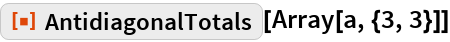Out=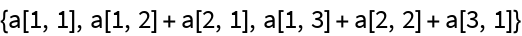Get the totals for a random matrix:

 In:=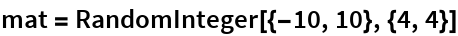Out=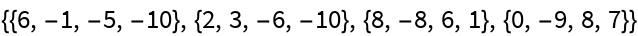In:=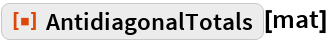Out=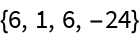### Properties and Relations

The direction of the antidiagonals:

 In:=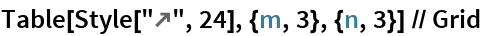Out=Pascal’s triangle:

 In:=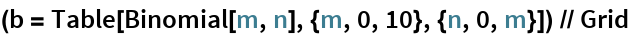Out=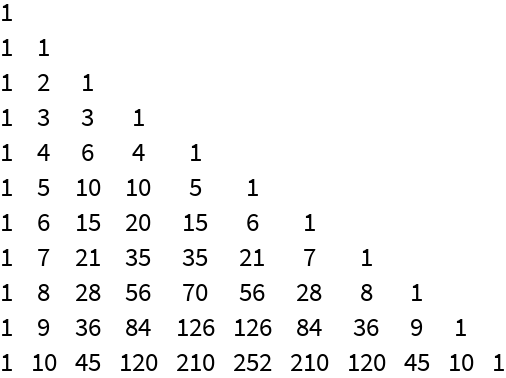The Fibonacci sequence:

 In:=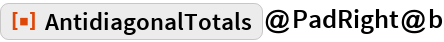Out=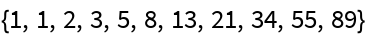In:=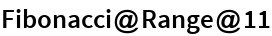Out=## Requirements

Wolfram Language 11.3 (March 2018) or above